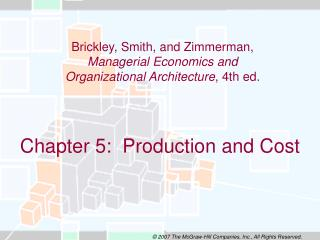DownloadDownload PresentationChapter 5: Production and Cost

# Chapter 5: Production and Cost

Download Presentation## Chapter 5: Production and Cost

- - - - - - - - - - - - - - - - - - - - - - - - - - - E N D - - - - - - - - - - - - - - - - - - - - - - - - - - -
##### Presentation Transcript

1. Chapter 5: Production and Cost Brickley, Smith, and Zimmerman, Managerial Economics and Organizational Architecture, 4th ed.

2. Production and costobjectives Students should be able to • Write a production function and distinguish between returns to scale and returns to a factor • Use isocosts and isoquants to illustrate production trade-offs • Employ short- and long-run cost curves to describe firm characteristics

3. Production functions A production function specifies maximum output from given inputs:

4. Empirical production functions • Cubic Q=a0+a1XY+a2X2Y+a3XY2+a4X3Y+a5XY3 • Cobb-Douglas Q = aXbYc log Q = log a + b log X + c log Y

5. Returns to scale Defined: The relation between output and a proportional variation of all inputs together Increasing returns to scale:Q=KL Decreasing returns to scale:Q=K1/3L1/3 Constant returns to scale:Q=K1/2L1/2

6. Returns to a factor Returns to a factor refer to the relation between output and variation in only one input • Total product • Average productQ/L • Marginal productQ/L

7. Returns to a factor Production function: Q=S1/2A1/2

8. Returns to a factora common case

9. Illustrating production choices with isoquants • Isoquants portray technical combination of inputs to produce a given level of output • Shape of isoquants indicates substitutability between inputs

10. Optimal input combinationisoquants

11. Differing input substitutabilityisoquants

12. Isocost lines • Isocosts portray combinations of inputs that entail the same cost • Isocosts change as input prices change

13. Isocost lines

14. Isocost lineschanges in input prices

15. Optimal input mix Where MPi is the marginal product of input i and Piis the price of input i.

16. Cost minimization

17. Optimal input mixinput price changes

18. Cost concepts • Total cost • relation between total cost and output • Marginal cost • change in total cost when output rises one unit • Average cost • total cost divided by total output • Opportunity cost • value of best alternative resource use

19. Cost curves

20. Short run versus long run • Short run • at least one input is fixed • cost curves are operating curves • Long run • all inputs are variable • cost curves are planning curves • Fixed costs--incurred even if firm produces nothing • Variable costs--change with the level of output

21. Short-run cost curves

22. Long-run average and marginal cost curves

23. Additional cost concepts • Minimum efficient scale • plant size at which long-run average cost first reaches its minimum point (Q*) • Economies of scope • cost of producing a joint set of products is less than cost of producing separately in separate firms • Learning curves • costs decline with production experience

24. Learning curve

25. Economies of scale versus learning effects

26. Profit maximization • A firm should increase output as long as marginal revenue exceeds marginal cost • A firm should not increase output if marginal cost exceeds marginal revenue • At the profit-maximizing level of output, MR=MC

27. Optimal output and changes in marginal cost

28. Factor demand Efficient production requires that MPi/Pi= MPj/Pj The reciprocals represent marginal cost Pi/MPi=Pj/MPj=MC At the optimum output level Pi/MPi=MR From which we derive the demand curve for input i Pi=MRMPi

29. Factor demand curve

30. Cost estimation • Effective management decisions should incorporate estimates of short- and long-run costs • Use regression analysis • Short-run costs may be approximately linear VC = a + bQ